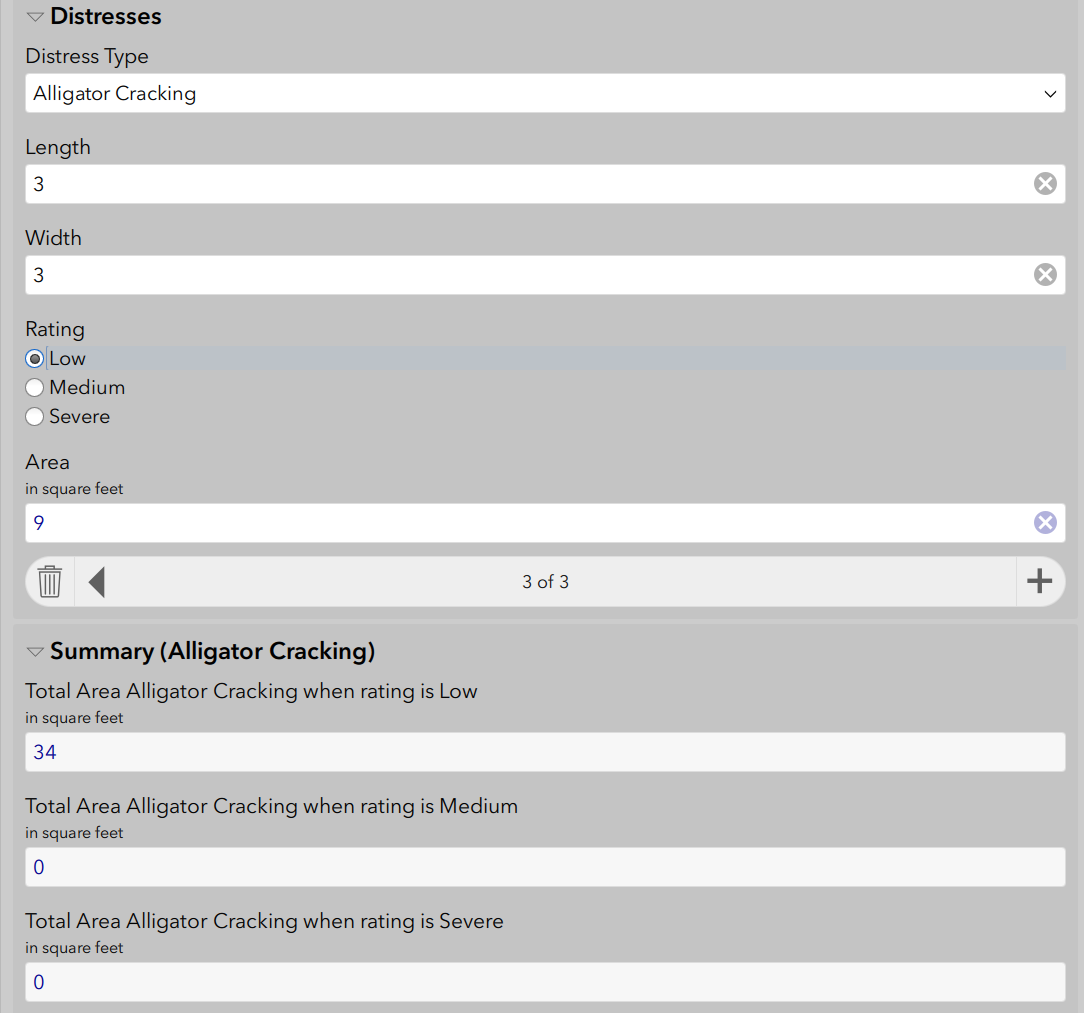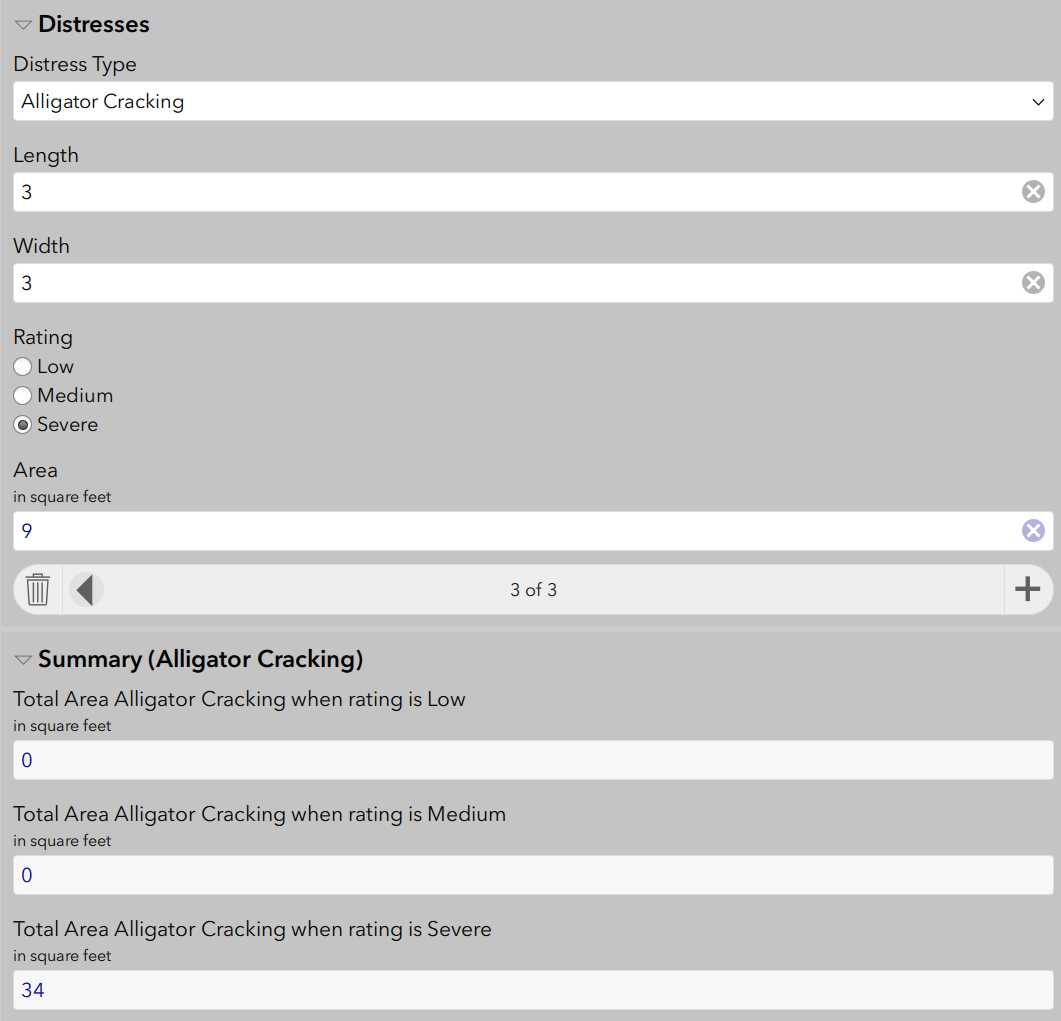# Survey123 - Repeat Calculation Sum Based on input

308
10
09-20-2019 12:45 PM
Highlighted
byMVP Regular Contributor

I am trying to get a summary of inputs based on 3 different questions. Using Repeat.

My questions are:

Area in sqft, Distress Type, Rating

Possible Distress types

Alligator Cracking, Bleeding, and Clock Cracking

Possible Ratings

Low, Med, High

Area is inputted

I hope to have an output of something like this:

 Rating Area of Alligator Cracking Low 20 Med 100 High 60 Total 180

 Rating Area of Bleeding Low 5 Med 6 High 7 Total 18

I am looking for a way to have the area increase after each repeat.

Thanks

Tags (2)
1 Solution

Accepted Solutions
Highlighted
byEsri Esteemed Contributor

Hi Rickey,

One way to this is, in the repeat, have a set of calculate questions for each of the category/value combinations you wish to create a sum for.  Each of these would have a formula using an if() function to check if the correct category was selected, and then put the value in if true and 0 if false.  For example, the 'Low, Alligator' category (lets call this low_allig) would look something

if(selected(\${Severity}, 'low') and selected(\${distress_type}, 'alligator'), \${sq_ft}, 0)

You then would have a question that uses the sum() function to add the values in the parent part of the form:

sum(\${low_allig})

10 Replies
Highlighted
byEsri Esteemed Contributor

Hi Rickey,

One way to this is, in the repeat, have a set of calculate questions for each of the category/value combinations you wish to create a sum for.  Each of these would have a formula using an if() function to check if the correct category was selected, and then put the value in if true and 0 if false.  For example, the 'Low, Alligator' category (lets call this low_allig) would look something

if(selected(\${Severity}, 'low') and selected(\${distress_type}, 'alligator'), \${sq_ft}, 0)

You then would have a question that uses the sum() function to add the values in the parent part of the form:

sum(\${low_allig})

Highlighted
byMVP Regular Contributor

The code works to sum up the current repeat but resets after each input. Is there a way to keep the total until the survey has been submitted?

Highlighted
Frequent Contributor II

Try the function once()

Highlighted
byMVP Regular Contributor

I can get the results to add but I want the numbers to always be displayed.

Right now when I use if(selected(\${Severity}, 'low') and selected(\${distress_type}, 'alligator'), \${sq_ft}, 0)

when severity low is not selected the total sq blanks out. I want the total displayed no matter the selected.

I have a formula for each severity

if(selected(\${Severity}, 'low') and selected(\${distress_type}, 'alligator'), \${sq_ft}, 0)

if(selected(\${Severity}, 'med') and selected(\${distress_type}, 'alligator'), \${sq_ft}, 0)

if(selected(\${Severity}, 'high') and selected(\${distress_type}, 'alligator'), \${sq_ft}, 0)

The output shows the total of distress type alligator no matter what the severity is. I want it to keep a tally of rating that shows at all times not just when the right rating is selected.

Each total should be 9 not 34Highlighted
Frequent Contributor II

Post your formula for sq_ft also.

Highlighted
byMVP Regular Contributor

My formula for sq_ft is

(\${width}*\${length})

Highlighted
Frequent Contributor II

Is the Summary section outside the repeat?  Seems like it.  If so then how would it know which width and length to use?

Are you maybe using sum or something?   I think you need to move the calcs into the repeat.  Then you can sum them in another field outside the repeat if you need to.

Highlighted
byMVP Regular Contributor

Yes I did have the summary outside the repeat.  I will try moving them in and see what happens.

Thanks!

Highlighted
Frequent Contributor II

Ok that makes sense then.  There is a width and height for each repeat.  Having it outside makes no sense then.